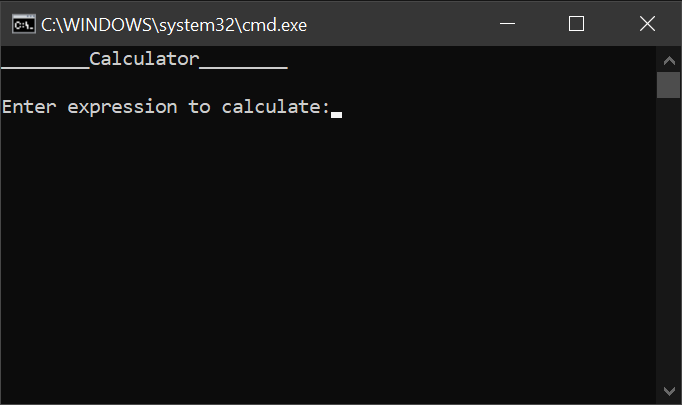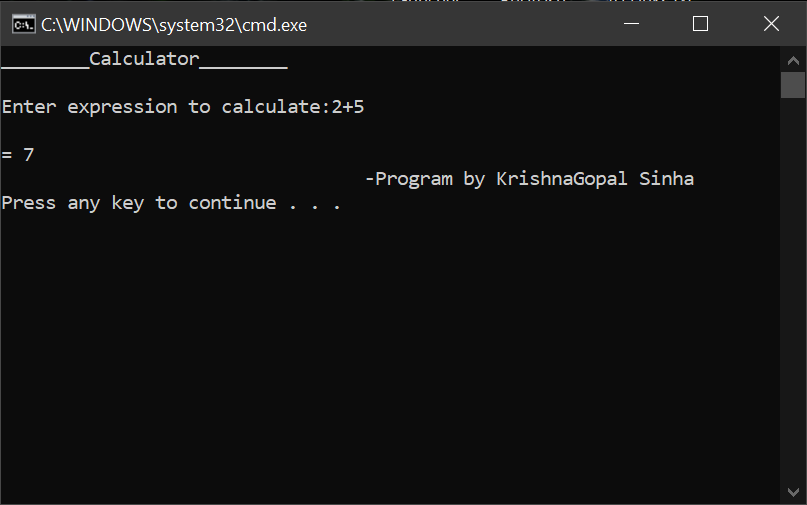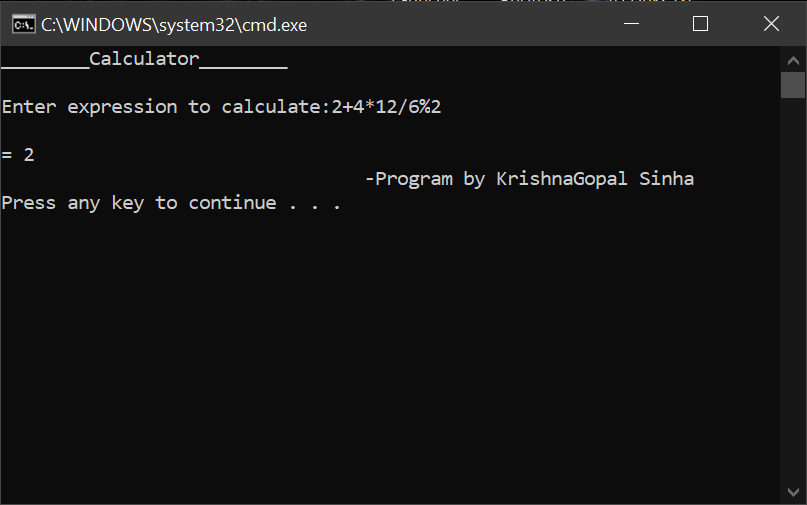# Calculator Program with Batch Scripting in Windows

• Difficulty Level : Basic
• Last Updated : 07 Sep, 2020

By using the Batch Scripting of command prompt one could even design a normal basic calculator that takes expressions and returns the result. Type the following script in notepad or any other text editor software:

```@echo off
:a
echo ________Calculator________
echo.
set /p expression= Enter expression to calculate:
set /a ans=%expression%
echo.
echo = %ans%
echo
pause
cls
goto a
```

After writing the above text in a text editor, save the file with the extension “ .bat “. To run the program, simply double-click on the saved file, the program will open and the code will get executed in Command Prompt. It should look something like:Welcome screen!

### Code Explanation and Test Cases:

• @echo off — signals Command Prompt to not display the commands (code).
• : a — the signals starting point of a loop.
• echo — same as print/println/printf/etc. Displays the specified message as output to the screen.
• echo. — will output a blank line.
• set — used to set value against a variable.
• set /p expression — /p signals that this is prompt. “Expression” is just a variable name.
• set /a ans – /a signal that variable has a numerical value. “ans” is the variable name.
• %ans% — calls the variable “ans”
• pause — will pause/ stop the program flow until the user will press a key (any key) and continue the program flow only when the users press a key (any).
• cls – clears the screen
• goto a- signals to go to the start point of the loop, effectively looping our calculator program so it can take in and calculate new expression.

Program Test Cases:

• The program can handle simple expression calculations like:Calculating Simple Expression

• And it can also handle complex expressions, such as:Calculating Complex Expression

Note:-

• The above program is calculating expressions by utilizing the calculator (that can perform simple arithmetic on 32-bit signed integers) that comes with Command Prompt.
• Since we are utilizing built-in calculator of Command Prompt, we can only perform following expression: + (addition), – (subtraction), * (multiplication),  / (divide), % (modulo).
My Personal Notes arrow_drop_up